# NCERT (intext and Exercise) Solution Science Class 9 Sound

In this page we have NCERT (intext and Exercise) Solution Science Class 9 Sound . Hope you like them and do not forget to like , social shar and comment at the end of the page.

## NCERT intext Solutions for Sound

Page 162

Question 1
How does the sound produce by a vibrating object in a medium reach your ear?
When an object vibrates, it vibrates the neighboring particles of the medium. These vibrating particles then vibrate to the adjacent particles After displacing the adjacent particle the first particle of medium comes back in its original position. This process continues in the medium till the sound reaches your ear.

Page 163
Question 2
Explain how sound is produced by your school bell.
When the bell continues to move forward and backward, it creates a series of compressions and rarefactions making production of sound.

Question 3
Why are sound waves called mechanical waves?
Sound waves needs material medium to propagate therefore, they are called mechanical waves.Sound waves propagate through a medium because of the interaction of the particles present in that medium.

Question 4
Suppose you and your friend are on the moon. Will you be able to hear any sound produced by your friend?
Sound waves needs a medium through which they can propagate. Since there is no material medium on the moon due to absence of atmosphere, you cannot hear any sound on the moon.

Page 166
Question 5
Which wave property determines (a) loudness, (b) pitch?
(a) Loudness is determined by Amplitude. The amplitude of a sound directly proportional to amplitude of wave. If the amplitude of a sound is large, then the sound produced will also be loud
(b) pitch is determined by Frequency. The pitch of a sound is proportional to its frequency. High pitched sounds have high frequency

Question 6
Guess which sound has a higher pitch: guitar or car horn?
Guitar has a higher pitch than car horn, because sound produced by the strings of guitar has high frequency than that of car horn. High the frequency higher is the pitch.

Question 7
What are wavelength, frequency, time period and amplitude of a sound wave?
 Wavelength The distance between two consecutive compressions or two consecutive rarefactions is known as the wavelength. Its SI unit is metre (m). Frequency The number of complete oscillations per second is known as the frequency of a sound wave. It is measured in hertz (Hz). Amplitude The maximum height reached by the crest or trough of a sound wave is called its amplitude.

Question 8
How are the wavelength and frequency of a sound wave related to its speed?
Speed, wavelength, and frequency of a sound wave are related by the following equation:
Speed (v) = Wavelength (λ) × Frequency (ν)
v =λ × ν

Question 9
Calculate the wavelength of a sound wave whose frequency is 220 Hz and speed is 440 m/s in a given medium.
Given in the question
Frequency of the sound wave(ν)= 220 Hz
Speed of the sound wave(v)= 440 m s-1
For a sound wave,
Speed (v) = Wavelength (λ) × frequency (ν)
v =λ × ν
or λ=v/ ν = 440 / 220 = 2m
So the wavelength of the sound wave is 2 m.

Question 10
A person is listening to a tone of 500 Hz sitting at a distance of 450 m from the source of the sound. What is the time interval between successive compressions from the source?
The time interval between two successive compressions is equal to the time period of the wave. This time period is reciprocal of the frequency of the wave and is given by the relation
T= 1 / Frequency = 1/ 500 = 0.002 s

Question 11
Distinguish between loudness and intensity of sound.
Intensity of a sound wave is defined as the amount of sound energy passing through a unit area per second. Loudness is a measure of the response of the ear to the sound. The loudness of a sound is defined by its amplitude. The amplitude of a sound decides its intensity, which in turn is perceived by the ear as loudness.

Page 167
Question 12
In which of the three media, air, water or iron, does sound travel the fastest at a particular temperature?
The speed of sound depends on the nature of the medium. Sound travels the fastest in solids. Its speed decreases in liquids and it is the slowest in gases. Therefore, for a given temperature, sound travels fastest in iron.

Page 168
Question 13
An echo returned in 3 s. What is the distance of the reflecting surface from the source, given that the speed of sound is 342 m s−1?
Given in the question
Speed of sound, v = 342 m /s
Echo returns in time, t = 3 s
Distance travelled by sound = v × t = 342 × 3 = 1026 m
Now sound has to travel a distance that is twice the distance of the reflecting surface and the source.
So the distance of the reflecting surface from the source= 1026 / 2 m = 513 m.

Page 169
Question 14
Why are the ceilings of concert halls curved?
Ceilings of concert halls are curved so that sound after reflection (from the walls) spreads uniformly in all directions.

Page 170
Question 15
What is the audible range of the average human ear?
The audible range of an average human ear lies between 20 Hz to 20,000 Hz.

Question 16
What is the range of frequencies associated with
(a) Infrasound?
(b) Ultrasound?
(a) Infrasound has frequencies less than 20 Hz.
(b) Ultrasound has frequencies more than 20,000 Hz.

Page 172
Question 17
A submarine emits a sonar pulse, which returns from an underwater cliff in 1.02 s. If the speed of sound in salt water is 1531 m/s, how far away is the cliff?
Time taken by the sonar pulse to return, t = 1.02 s
Speed of sound in salt water, v = 1531 m/
Distance travelled by Sound = Speed of sound x Time taken
Distance travelled by Sound = 1.02 x 1531 = 1561.62 m
Distance travelled by the sonar pulse during its transmission and reception in water = 2 x Actual distance = 2d
Actual Distance, d= Distance of the cliff from the submarine/2
= 1561/2
= 780.31 m

## NCERT Exercise Solutions for Sound

Question 18
What is sound and how is it produced?
Sound is a form of energy which gives the sensation of hearing. It is produced by the vibrations caused in air by vibrating objects.

Question 19
Describe with the help of a diagram, how compressions and rarefactions are produced in air near a source of sound.
When a vibrating body moves forward, it create a region of high pressure in its vicinity. This region of high pressure is known as compressions. When it moves backward, it creates a region of low pressure in its vicinity. This region is known as a rarefaction. As the body continues to move forward and backwards, it produces a series of compressions and rarefactions. This is shown in below figure.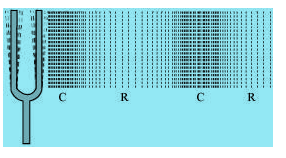Question 20
Cite an experiment to show that sound needs a material medium for its propagation.
Step 1:
Take an electric bell and an air tight glass bell jar connected to a vacuum pump.
Step 2:
Suspend the bell inside the jar, and press the switch of the bell.
Step 3:You will be able to hear the bell ring.
Step 4:Now pump out the air from the glass jar.
Step 5: Now press the switch of the bell,The sound of the bell will become fainter and after some time, the sound will not be heard. This is so because almost all air has been pumped out.
This shows that sound needs a material medium to travel.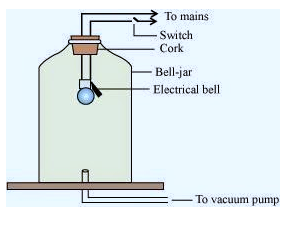Question 21
Why is sound wave called a longitudinal wave?
Sound wave is called longitudinal wave because it is produced by compressions and rarefactions in the air. The air particles vibrate parallel to the direction of propagation.

Question 22
Which characteristics of the sound helps you to identify your friend by his voice while sitting with others in a dark room?
The quality or timber of sound enables us to identify our friend by his voice.

Question 23
Flash and thunder are produced simultaneously. But thunder is heard a few seconds after the flash is seen, why?
The speed of sound (344 m/s)is very less than the speed of light(3 x 108 m/s). Sound of thunder takes more time to reach the Earth as compared to light. Hence, a flash is seen before we hear a thunder.

Question 24
A person has a hearing range from 20 Hz to 20 kHz. What are the typical wavelengths of sound waves in air corresponding to these two frequencies? Take the speed of sound in air as 344 m s−1.

For a sound wave,
Speed = Wavelength x Frequency=λ x ν
Speed of sound in air = 344 m/s (Given)
(i) For,ν= 20 Hz
λ1= v/ν = 344/20 = 17.2 m

(ii) For, ν= 20000 Hz
λ2= v/ν = 344/20000 = 0.172 m

Hence, for humans, the wavelength range for hearing is 0.0172 m to 17.2 m.

Question 25
Two children are at opposite ends of an aluminium rod. One strikes the end of the rod with a stone. Find the ratio of times taken by the sound wave in air and in aluminium to reach the second child.
Velocity of sound in air= 346 m/s
Velocity of sound wave in aluminium= 6420 m/s
Let length of aluminium rod is L
Time taken for sound wave in air(tair)= L / Velocity in air
Time taken for sound wave in Aluminium(taluminium)= L / Velocity in aluminium

Therefore, tair / taluminium = Velocity inaluminium/ Velocity in air = 6420 / 346 = 18.55 : 1

Question 26
The frequency of a source of sound is 100 Hz. How many times does it vibrate in a minute?
It is given
Frequency = 100 Hz
By definition of frequency, this means the source of sound vibrates 100 times in one second.
So number of vibrations in 1 minute, i.e. in 60 seconds = 100 x 60 = 6000 times.

Question 27
Does sound follow the same laws of reflection as light does? Explain.
Sound follows the same laws of reflection as light does. The laws are
(i) The incident sound wave and the reflected sound wave make the same angle with the normal to the surface at the point of incidence.
(ii) the incident sound wave, the reflected sound wave, and the normal to the point of incidence all lie in the same plane.

Question 28
When a sound is reflected from a distant object, an echo is produced. Let the distance between the reflecting surface and the source of sound production remains the same. Do you hear echo sound on a hotter day?
An echo is heard when the time for the reflected sound is heard after 0.1 s
Time Taken= Total Distance / Velocity
On a hotter day, the velocity of sound is more. If the time taken by echo is less than 0.1 sec it will not be heard.

Question 29
Give two practical applications of reflection of sound waves.
Two practical applications of reflection of sound waves are:
(1) Reflection of sound is used to measure the distance and speed of underwater objects. This method is known as SONAR.
(2) Working of a stethoscope is also based on reflection of sound. In a stethoscope, the sound of the patient's heartbeat reaches the doctor's ear by multiple reflection of sound.

Question 30
A stone is dropped from the top of a tower 500 m high into a pond of water at the base of the tower. When is the splash heard at the top? Given, g = 10 m s−2 and speed of sound = 340 m s−1.
Time when the splash is heard will the sum of time taken by stone to fall in the pond plus then time taken the sound to reach the top of the tower
So calculating them separately
Height of the tower, s = 500 m
Acceleration due to gravity, g = 10 m s−2
Initial velocity of the stone, u = 0 (since the stone is initially at rest)
Time taken by the stone to fall to the base of the tower, t
According to the second equation of motion
S= ut + (1/2)gt2
Substituting the values of s,u and g, we get
t=10 s

Velocity of sound, v = 340 m s−1
Now, time taken by the sound to reach the top from the base of the tower, T= Height/Velocity of sound
=500 / 340 = 1.47 s
Therefore, the splash is heard at the top after time
=t + T= 10 + 1.47 = 11.47 s.

Question 31
A sound waves travels at a speed of 339 m/s. If its wavelength is 1.5 cm, what is the frequency of the wave? Will it be audible?
Given
Speed of sound, v= 339 m/s
Wavelength of sound,λ= 1.5 cm = 0.015 m
Now we know that
Speed of sound = Wavelength x Frequency= λ x v
or v= v /λ = 339 / 0.015 = 22600 Hz
As we know that The frequency range of audible sound for humans lies between 20 Hz to 20,000 Hz, Since the frequency of the given sound is more than 20,000 Hz, it is not audible.

Question 32
What is reverberation? How can it be reduced?
The repeated multiple reflections of sound in any big enclosed space is known as reverberation.
The reverberation can be reduced by covering the ceiling and walls of the enclosed space with sound absorbing materials, such as fibre board, loose woollens, etc.

Question 33
What is loudness of sound? What factors does it depend on?
The effect produced in the brain by the sound of different frequencies is called loudness of sound.
Loudness depends on the amplitude of vibrations. In fact, loudness is proportional to the square of the amplitude of vibrations.

Question 34
Explain how bats use ultrasound to catch a prey.
Bats produce high-pitched ultrasonic squeaks. These high-pitched squeaks are reflected by objects such as preys and returned to the bat's ear. This allows a bat to know the distance of his prey.

Question 35
How is ultrasound used for cleaning?
Objects to be cleansed are put in a cleaning solution and ultrasonic sound waves are passed through that solution. The high frequency of these ultrasound waves detaches the dirt from the objects.

Question 36
Explain the working and application of a sonar.
SONAR is SOund Navigation And Ranging. It is a technique used to measure the depth of the sea, locate the sunken ships or icebergs and submarines.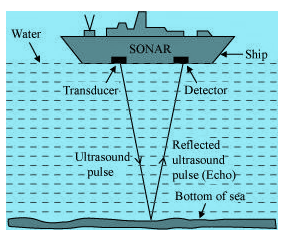A beam of ultrasonic sound is produced and transmitted by the transducer (it is a device that produces ultrasonic sound) of the SONAR, which travels through sea water. The echo produced by the reflection of this ultrasonic sound is detected and recorded by the detector, which is converted into electrical signals. The distance (d) of the under-water object is calculated from the time (t) taken by the echo to return with speed (v) is given by 2d = v × t. This method of measuring distance is also known as ‘echo-ranging’.

Question 37
A sonar device on a submarine sends out a signal and receives an echo 5 s later. Calculate the speed of sound in water if the distance of the object from the submarine is 3625 m.
Given
Time taken to hear the echo, t= 5 s
Distance of the object from the submarine, d= 3625 m
Now we know Total distance travelled by the sonar waves during the transmission and reception in water= 2d
So Velocity of sound in water will be given by
v=Distance /time = 2d / t = 2 x 3625 / 5 = 1450 m/s

Question 38
Explain how defects in a metal block can be detected using ultrasound.
Defects in metal blocks do not allow ultrasound to pass through them and they are reflected back. This fact is used to detect defects in metal blocks. Ultrasound is passed through one end of a metal block and detectors are placed on the other end. The defective part of the metal block does not allow ultrasound to pass through it. As a result, it will not be detected by the detector. Hence, defects in metal blocks can be detected using ultrasound.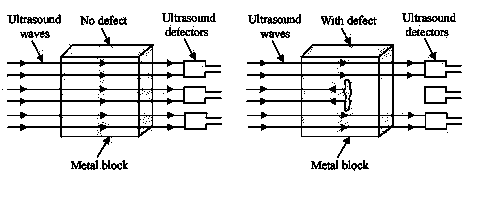Question 39
Explain how the human ear works.
The human ear consists of three parts – the outer ear, middle ear and inner ear. The processing done by each part is explained below
 Outer ear Middle ear Inner ear This is also called ‘pinna’. It collects the sound from the surrounding and directs it towards auditory canal. The sound reaches the end of the auditory canal where there is a thin membrane called eardrum or tympanic membrane. The sound waves set this membrane to vibrate. These vibrations are amplified by three small bones- hammer, anvil and stirrup These vibrations reach the cochlea in the inner ear and are converted into electrical signals which are sent to the brain by the auditory nerve, and the brain interprets them as sound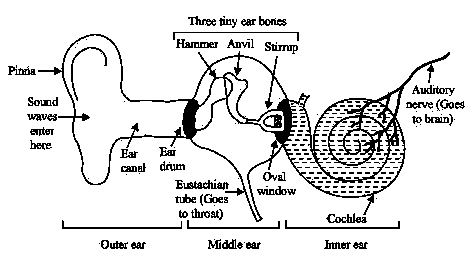## Summary

1. NCERT (intext and Exercise) Solution Science Class 9 Sound Chapter 12 has been prepared by Expert with utmost care. If you find any mistake.Please do provide feedback on mail. You can download the solutions as PDF in the below Link also